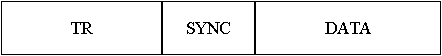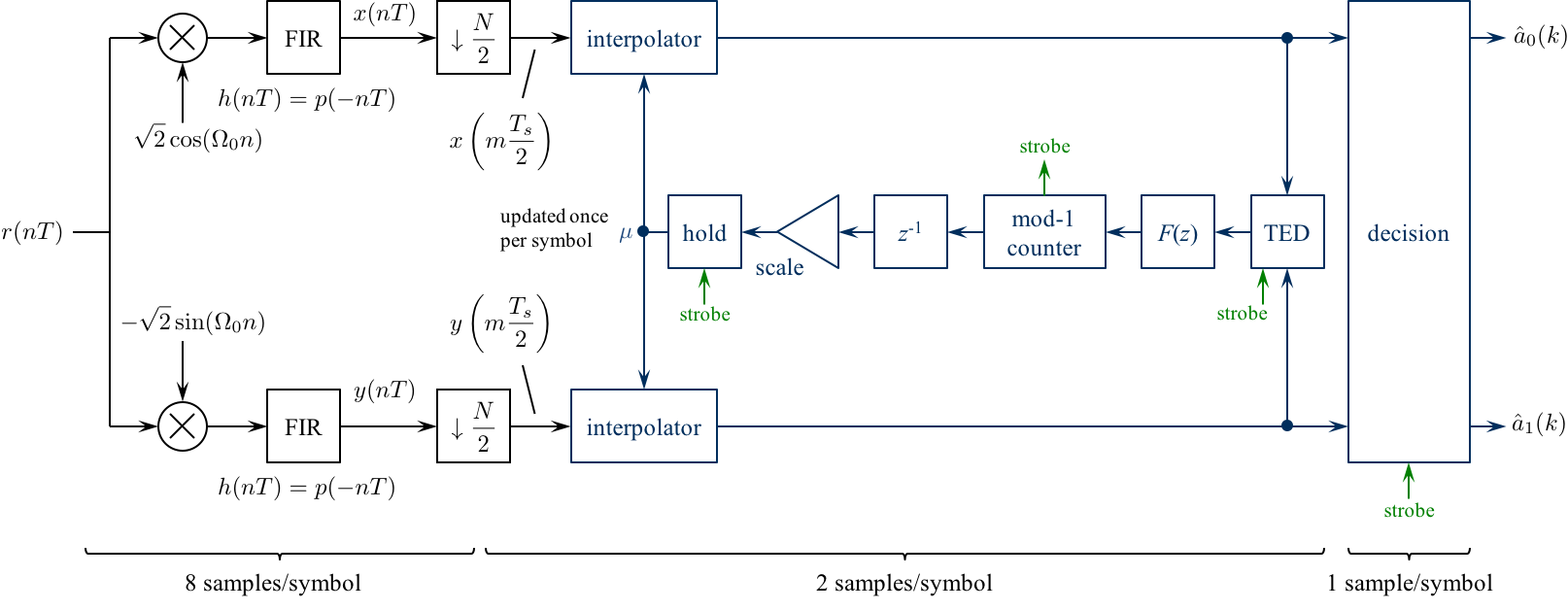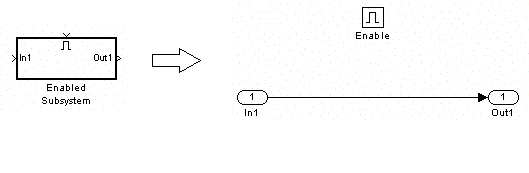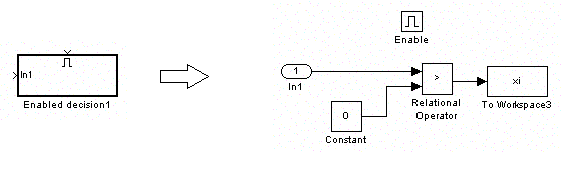# Digital Communications: A Discrete-Time Approachby Michael Rice# Symbol Timing Synchronization for QPSK

## Introduction

The previous Carrier Phase Synchronization for QPSK Using Differential Encoding/Decoding assumed the phase of carrier and the symbol clock were known. That is, you knew the phase of the unmodulated carrier and you knew where the symbols began and ended. Unfortunately, this is not the case in practice -- in a real system the detector knows neither the carrier phase nor the bit (or symbol timing). The bit (or symbol) timing information must be extracted from the received samples. This is accomplished using a bit (or symbol) timing synchronizer. In this exercise, you are given the carrier phase (i.e., it is known), but in contrast, the bit (or symbol) timing is not known.

In this exercise, you will design a symbol timing synchronizer for QPSK based on the PLL. There are three sample rates in play in this design, so you must be careful in assigning sample times to the Simulink blocks. The detector, with its accompanying bit timing synchronizer, will be used to process the modulated sampled in the file qpsktrdata.mat.

## Textbook References

M-ary QAM: Section 5.3 (pp. 223 - 240), discrete-time realizations: Section 5.3.2 (pp. 237 - 240), partial response pulse shapes: Section A.2 (pp. 629 - 646), general discussion of timing synchronization: Section 8.1 (pp. 404 - 406), discrete-time techniques for symbol timing synchronization for binary PAM: Section 8.4 (pp. 413 - 461), discrete-time techniques for symbol timing synchronization for BPSK: Section 8.5 (pp. 461 - 464).

## Specificationsnormalized sample rate: 8 samples/bit normalized carrier frequency: 0.3 cycles/sample carrier phase 0 degrees average energy: 1 pulse shape: SRRC (50% excess bandwidth, span = 12 symbols) symbol clock offset: unknown!! input file qpsktrdata.mat packet format: TR = 1000 bits (you don't need to know what they are) SYNC = 0 1 0 1 0 1 0 1 0 1 0 1 0 1 0 1 DATA = 2842 bits## Preliminary Design

### Design the Detector

Design the detector, shown below, using blocks from the Simulink, DSP System, and Communications System Toolboxes.Design the loop filter to create a second order loop to the following specifications: closed-loop equivalent noise bandwidth = 0.01 (normalized to the bit rate), damping factor = 0.7071.

### Design Notes

1. Because I plan on using the early-late detector for the timing error detector (TED) and the early-late TED operates at 2 samples/symbol, the matched filter output output is downsampled by 4. The matched filter and its input operate at 8 samples/symbol: the downsampled sequence is at 2 samples/symbol.

2. Use a Farrow interpolator. You can use either the piecewise-parabolic version (see the top of Figure 8.4.17 on p. 437) or the cubic version (see the bottom of Figure 8.4.18 on p. 438).

3. The strobe is used as an enable signal for the TED, decision, and mu update. he strobe signal is derived from the decrementing modulo-1 counter. Use the underflow condition in the decrementing mod-1 counter as the strobe.

4. You need to create an "enabled" version of the early-late TED. When the strobeis high, the TED output is given by equation (8.34) on page 420. When the strobe is low, the TED output is zero.

5. For interpolation control, use the decrementing modulo-1 counter described in Section 8.4.3 (pp. 441 - 444).

6. The "enabled hold" block outputs the previous value when the strobe is low, or passes the input to the output when the strobe is high. This block is needed because the fractional interval is updated by the mod-1 counter only once per symbol, but the interpolator operates at 2 samples/symbol. The hold operation ensures that the interpolator is using the proper value for the fractional interval.

Simulink provides a skeletal enabled subsystem:

Simulink -> Ports & Subsystems -> Enabled Sybsystem

The default enabled subsystem is shown belowThe subsystem consists of a simple wire with the enable icon above it. This means the wire is only active when the enable signal exceeds a predefined threshold (double click on the enable block to set the threshold). When the enable signal is below the predefined threshold, nothing happens and the output holds its value.

7. The decision subsystem is also enabled. This is because decisions should be made on every other interpolator output on average. The enable signal indicates which interpolator outputs represent the desired signal space projections. An example of the enabled decision subsystem for binary PAM is shown below## Exercise

1. Incorporate the symbol timing synchronization PLL into a QPSK detector.

2. For the detector input, use the From File block and set the Filename to qpsktrdata.mat and the sample time to 1.

3. Set the simulation parameters as follows:
 Simulation Time
 Start Time: 0.0 Stop Time: (2429+13)*8
Solver Options
 Type: Fixed-step Solver: discrete (no continuous states) Fixed step size: auto